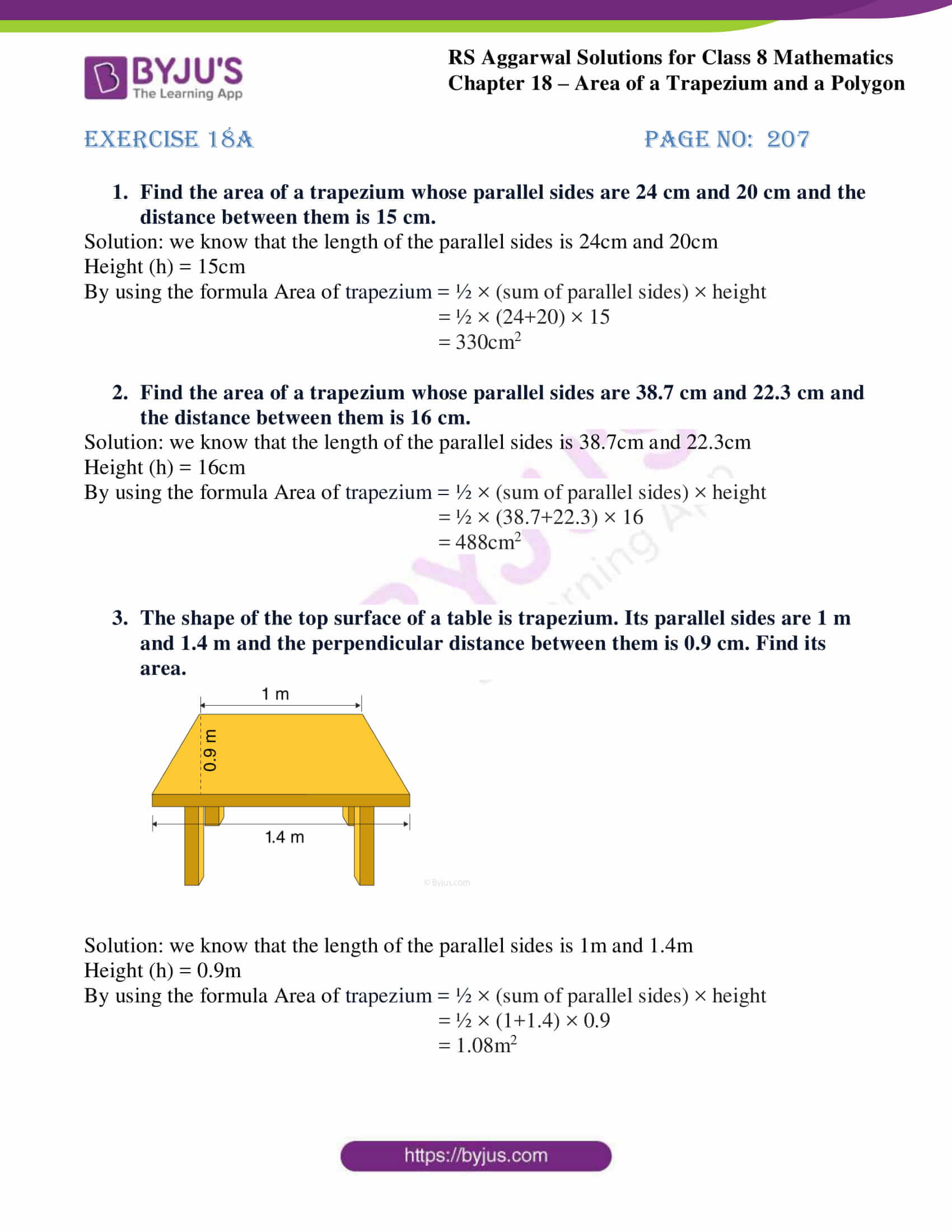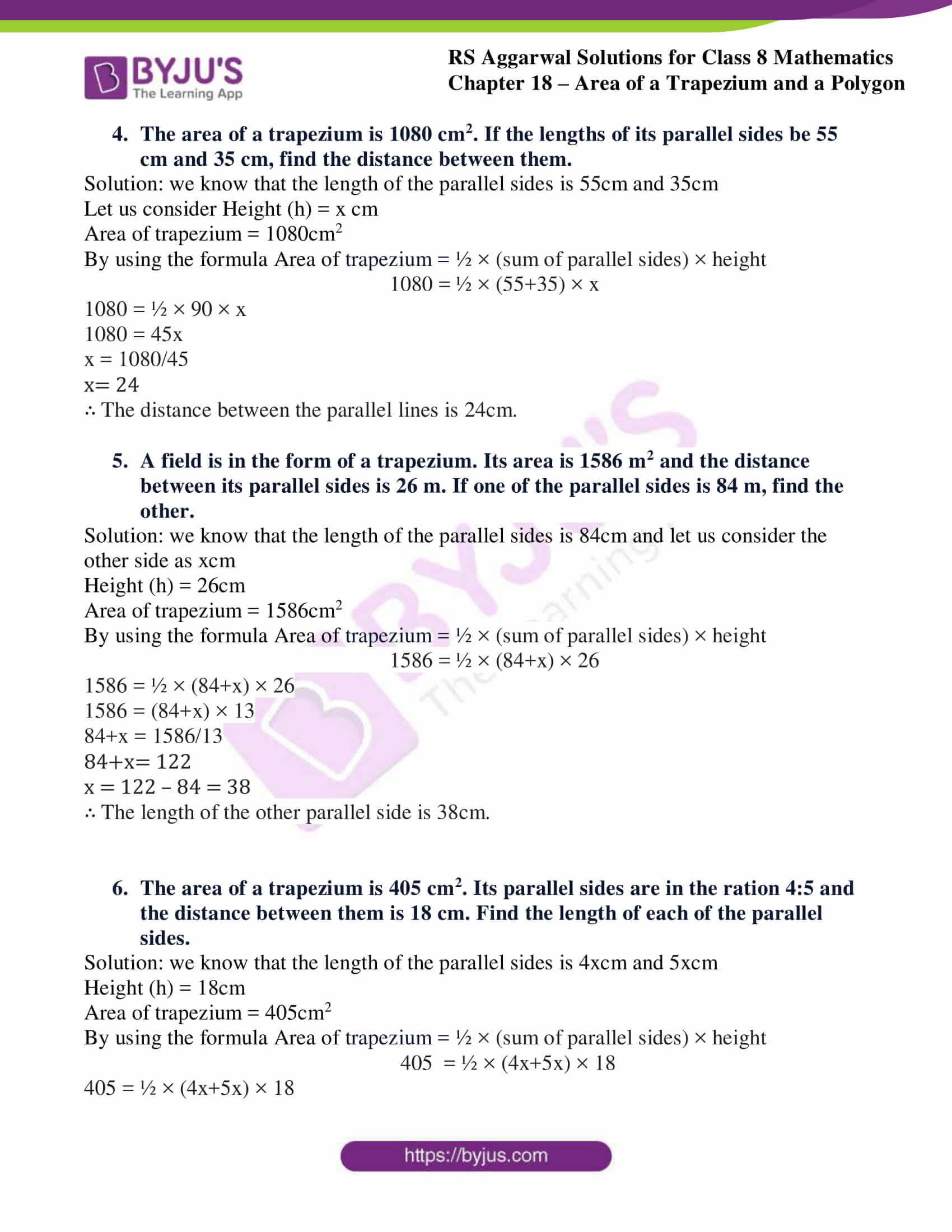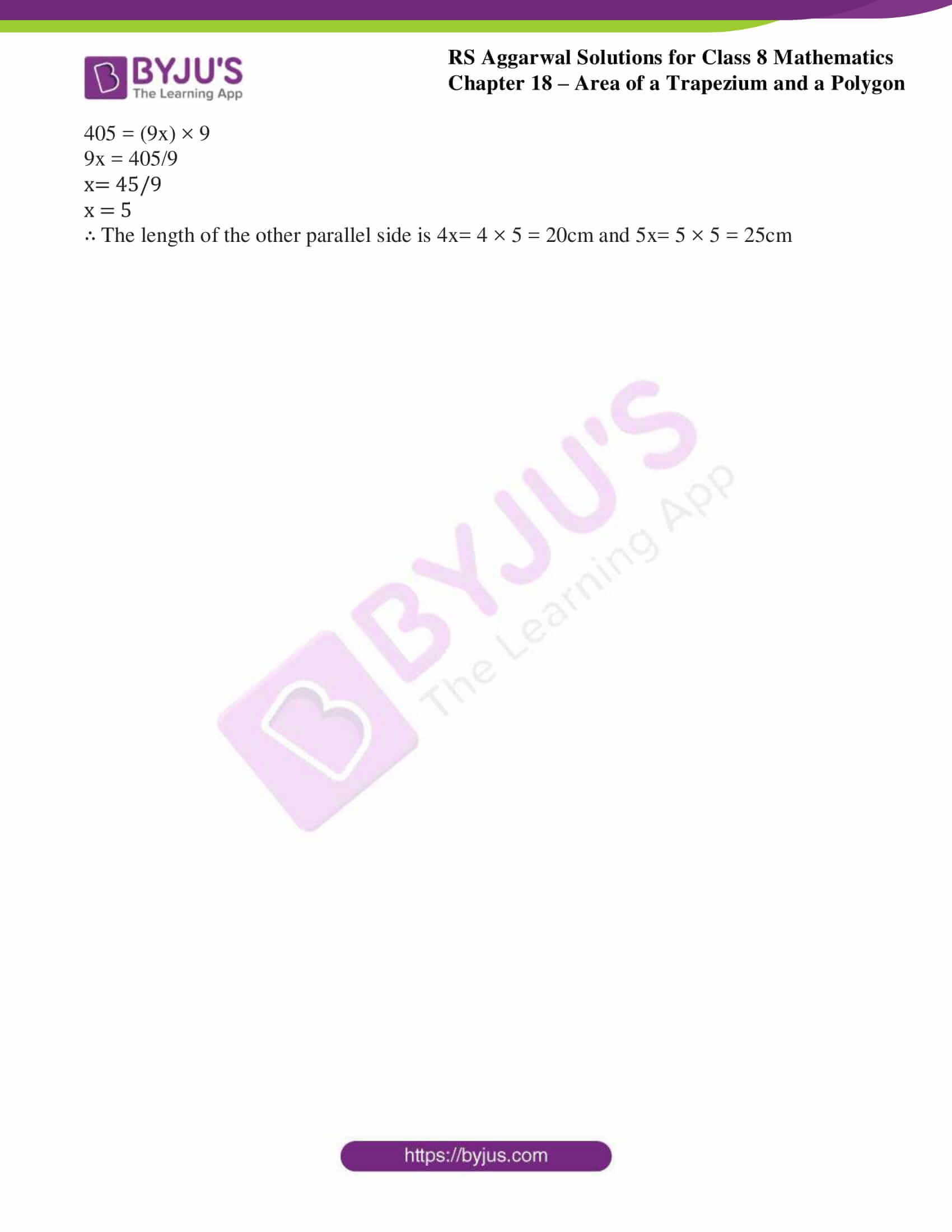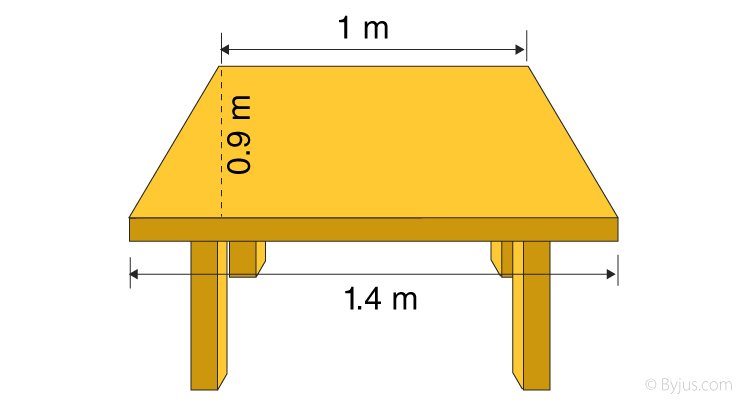# RS Aggarwal Solutions for Class 8 Chapter 18 - Area of a Trapezium and a Polygon Exercise 18A

BYJU’S expert team has solved the RS Aggarwal Solutions for Class 8 Maths Chapter 18- Exercise 18A, Area of a Trapezium and a Polygon, students can download from the links provided below. Solutions are solved in such a way that it is helpful in scoring high marks in the exams. In Exercise 18A of RS Aggarwal Class 8 Maths, we mainly find the area of a Trapezium. A Trapezium is a quadrilateral having one pair of parallel opposite sides.

## Download PDF of RS Aggarwal Solutions for Class 8 Maths Chapter 18- Exercise 18A### Access Answers to RS Aggarwal Solutions for Class 8 Maths Chapter 18- Area of a Trapezium and a Polygon Exercise 18A

1. Find the area of a trapezium whose parallel sides are 24 cm and 20 cm and the distance between them is 15 cm.

Solution: we know that the length of the parallel sides is 24cm and 20cm

Height (h) = 15cm

By using the formula Area of trapezium = ½ × (sum of parallel sides) × height

= ½ × (24+20) × 15

= 330cm2

2. Find the area of a trapezium whose parallel sides are 38.7 cm and 22.3 cm and the distance between them is 16 cm.

Solution: we know that the length of the parallel sides is 38.7cm and 22.3cm

Height (h) = 16cm

By using the formula Area of trapezium = ½ × (sum of parallel sides) × height

= ½ × (38.7+22.3) × 16

= 488cm2

3. The shape of the top surface of a table is trapezium. Its parallel sides are 1 m and 1.4 m and the perpendicular distance between them is 0.9 cm. Find its area.Solution: we know that the length of the parallel sides is 1m and 1.4m

Height (h) = 0.9m

By using the formula Area of trapezium = ½ × (sum of parallel sides) × height

= ½ × (1+1.4) × 0.9

= 1.08m2

4. The area of a trapezium is 1080 cm2. If the lengths of its parallel sides be 55 cm and 35 cm, find the distance between them.

Solution: we know that the length of the parallel sides is 55cm and 35cm

Let us consider Height (h) = x cm

Area of trapezium = 1080cm2

By using the formula Area of trapezium = ½ × (sum of parallel sides) × height

1080 = ½ × (55+35) × x

1080 = ½ × 90 × x

1080 = 45x

x = 1080/45

x= 24

∴ The distance between the parallel lines is 24cm.

5. A field is in the form of a trapezium. Its area is 1586 m2 and the distance between its parallel sides is 26 m. If one of the parallel sides is 84 m, find the other.

Solution: we know that the length of the parallel sides is 84cm and let us consider the other side as xcm

Height (h) = 26cm

Area of trapezium = 1586cm2

By using the formula Area of trapezium = ½ × (sum of parallel sides) × height

1586 = ½ × (84+x) × 26

1586 = ½ × (84+x) × 26

1586 = (84+x) × 13

84+x = 1586/13

84+x= 122

x = 122 – 84 = 38

∴ The length of the other parallel side is 38cm.

6. The area of a trapezium is 405 cm2. Its parallel sides are in the ration 4:5 and the distance between them is 18 cm. Find the length of each of the parallel sides.

Solution: we know that the length of the parallel sides is 4xcm and 5xcm

Height (h) = 18cm

Area of trapezium = 405cm2

By using the formula Area of trapezium = ½ × (sum of parallel sides) × height

405 = ½ × (4x+5x) × 18

405 = ½ × (4x+5x) × 18

405 = (9x) × 9

9x = 405/9

x= 45/9

x = 5

∴ The length of the other parallel side is 4x= 4 × 5 = 20cm and 5x= 5 × 5 = 25cm

## RS Aggarwal Solutions for Class 8 Maths Chapter 18- Exercise 18A

Exercise 18A of RS Aggarwal Class 8, Area of a Trapezium and a Polygon. This exercise mainly deals with questions related to finding the Area of a Trapezium whose sides or altitudes would be provided.

Students are suggested to practice the problems on a regular basis which will help them excel in their exams and increase their overall percentage. Practicing as many times as possible helps in building time management skills and also boosts the confidence level to achieve high marks.